## How to Calculate and Solve for P(A and B) | Probability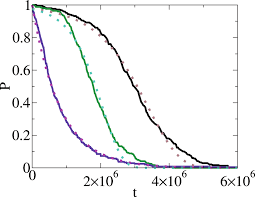The image above represents P(A and B).

To compute for P(A and B), four essential parameters are needed and these parameters are xA, NA, xB and NB.

The formula for calculating P(A and B):

P(A and B) = P(A) x P(B)

Where;

P(A) =  xANA
P(B) = xBNB

Let’s solve an example;
Find the P(A and B) when xA is 2, NA is 4, xB is 5 and NB is 8.

This implies that;

xA = 2
NA = 4
xB = 5
NB = 8

P(A and B) = P(A) x P(B)
P(A and B) = xANA x xBNB
P(A and B) = 24 x 58
P(A and B) = (2)(5)(4)(8)
P(A and B) = 1032
Divinding the numerator and denominator by 2
P(A and B) = 516
P(A and B) = 0.312

Therefore, the P(A and B) is 0.312.

## How to Calculate and Solve for P(A or B) | Probability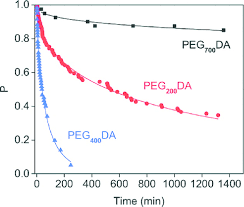The image above represents P(A or B).

To compute for P(A or B), four essential parameters are needed and these parameters are xA, NA, xB and NB.

The formula for calculating P(A or B):

P(A or B) = P(A) + P(B)

Where;

P(A) =  xANA
P(B) = xBNB

Let’s solve an example;
Find the P(A or B) when xA is 9, NA is 11, xB is 6 and NB is 12.

This implies that;

xA = 9
NA = 11
xB = 6
NB = 12

P(A or B) = P(A) + P(B)
P(A or B) = xANA + xBNB
P(A or B) = 911 + 612
P(A or B) = 9(12) + 6(11)(11)(12)
P(A or B) = 108 + 66132
P(A or B) = 174132
Dividing the numerator and denominator by 6
P(A or B) = 2922
P(A or B) = 1.318

Therefore, the P(A or B) is 1.318.

## How to Calculate and Solve for P(not A) | Probability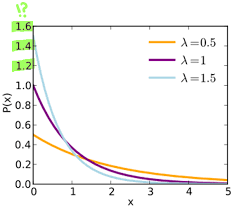The image above represents P(not A).

To compute for P(not A), two essential parameters are needed and these parameters are Number of Times in Which Event A Can Occur (x) and Total Number of All Possible Outcomes (N).

The formula for calculating P(not A):

P(not A) = (N – x)N

Where;

x = Number of Times in Which Event A can Occur
N = Total Number of All Possible Outcomes

Let’s solve an example;
Find the P(not A) when the number of times in which event A can occur is 7 and the total number of all possible outcomes is 11.

This implies that;

x = Number of Times in Which Event A can Occur = 7
N = Total Number of All Possible Outcomes = 11

P(not A) = (N – x) N
P(not A) = (11 – 7) 11
P(not A) = 4 11
P(not A) = 0.36

Therefore, the P(not A) is 0.36.

## How to Calculate and Solve for P(A) | Probability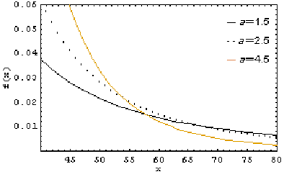The image above represents P(A).

To compute for P(A), two essential parameters are needed and these parameters are Number of Times in Which Event A Can Occur (x) and Total Number of All Possible Outcomes (N).

The formula for calculating P(A):

P(A) = x N

Where;

x = Number of Times in Which Event A can Occur
N = Total Number of All Possible Outcomes

Let’s solve an example;
Find the P(A) when the number of times in which event A can occur is 10 and the total number of all possible outcomes is 22.

This implies that;

x = Number of Times in Which Event A can Occur = 10
N = Total Number of All Possible Outcomes = 22

P(A) = x N
P(A) = 10 22
Dividing the numerator and denominator by 2
P(A) = 5 11
P(A) = 0.45

Therefore, the P(A) is 0.45.

## How to Calculate and Solve for Certainty | Probability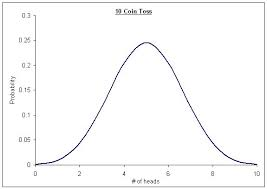The image above represents Certainty.

To compute for certainty, one essential parameter is needed and this parameter is Total Number of All Possible Outcomes (N).

The formula for calculating Certainty:

P(A) = x N = N N

Where;

P(A) = Certainty
N = Total Number of All Possible Outcomes = 2

Let’s solve an example;
Given that the total number of all possible outcomes is 2. Find the Certainty?

This implies that;

N = Total Number of All Possible Outcomes = 2

P(A) = x N = N N
P(A) = 2 2
P(A) = 1

Therefore, P(certainty) is 1.

## How to Calculate and Solve for Multiple Samples | Probability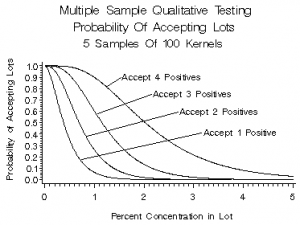The image above represents multiple samples.

To compute for multiple samples, one essential parameter is needed and this parameter is Number of Trials (N).

If Number of Trials (N) is 4.

N      x

1      10
2       5
3       2
4       1

The multiple samples will be calculated:

N       x      Cum x        Running average

1       10        10                 10.0
2        5         15                 7.50
3        2         17                 5.67
4        1         18                 4.50

Nickzom Calculator – The Calculator Encyclopedia is capable of calculating the multiple samples.

To get the answer and workings of the multiple samples using the Nickzom Calculator – The Calculator Encyclopedia. First, you need to obtain the app.

You can get this app via any of these means:

To get access to the professional version via web, you need to register and subscribe for NGN 1,500 per annum to have utter access to all functionalities.
You can also try the demo version via https://www.nickzom.org/calculator

Apple (Paid) – https://itunes.apple.com/us/app/nickzom-calculator/id1331162702?mt=8
Once, you have obtained the calculator encyclopedia app, proceed to the Calculator Map, then click on Probability under Mathematics.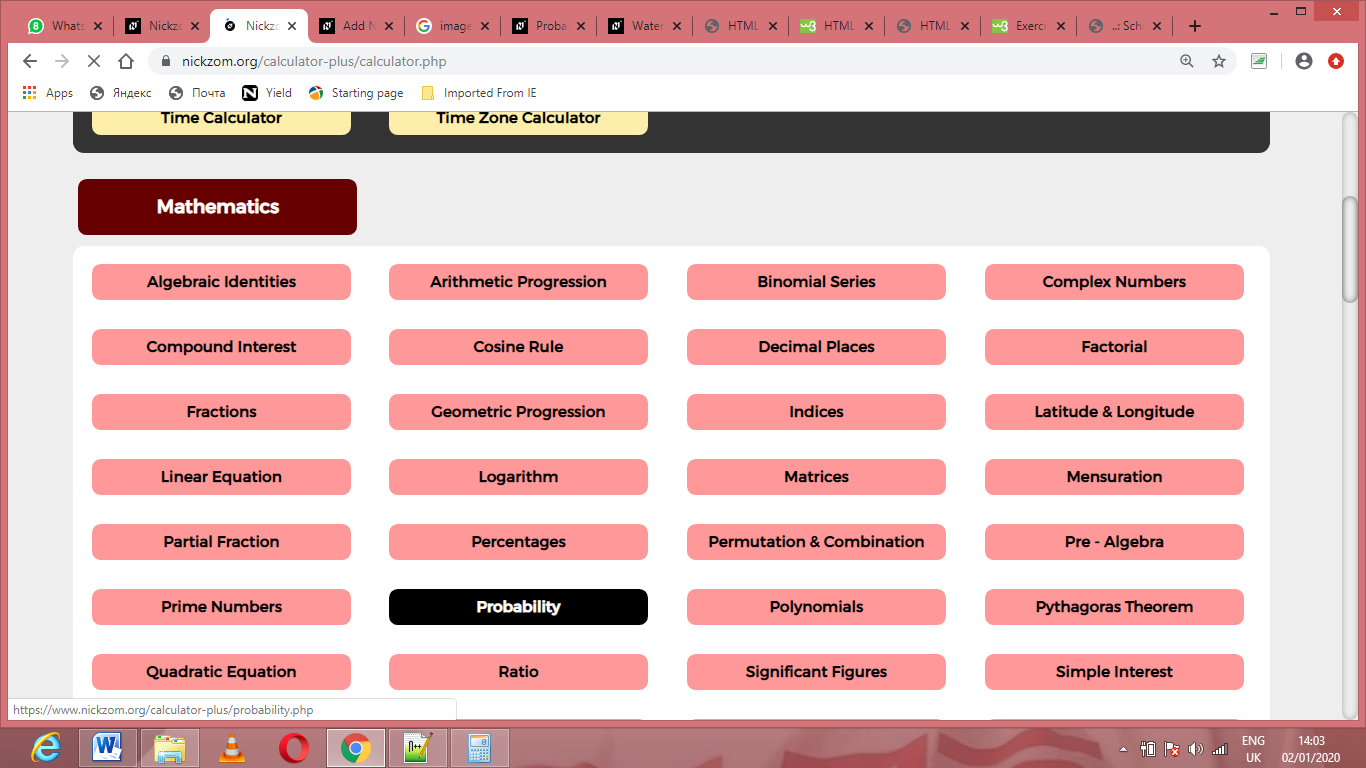Now, Click on Multiple Samples under ProbabilityThe screenshot below displays the page or activity to enter your value, to get the answer for the multiple samples according to the respective parameter which is the Number of Trial (N).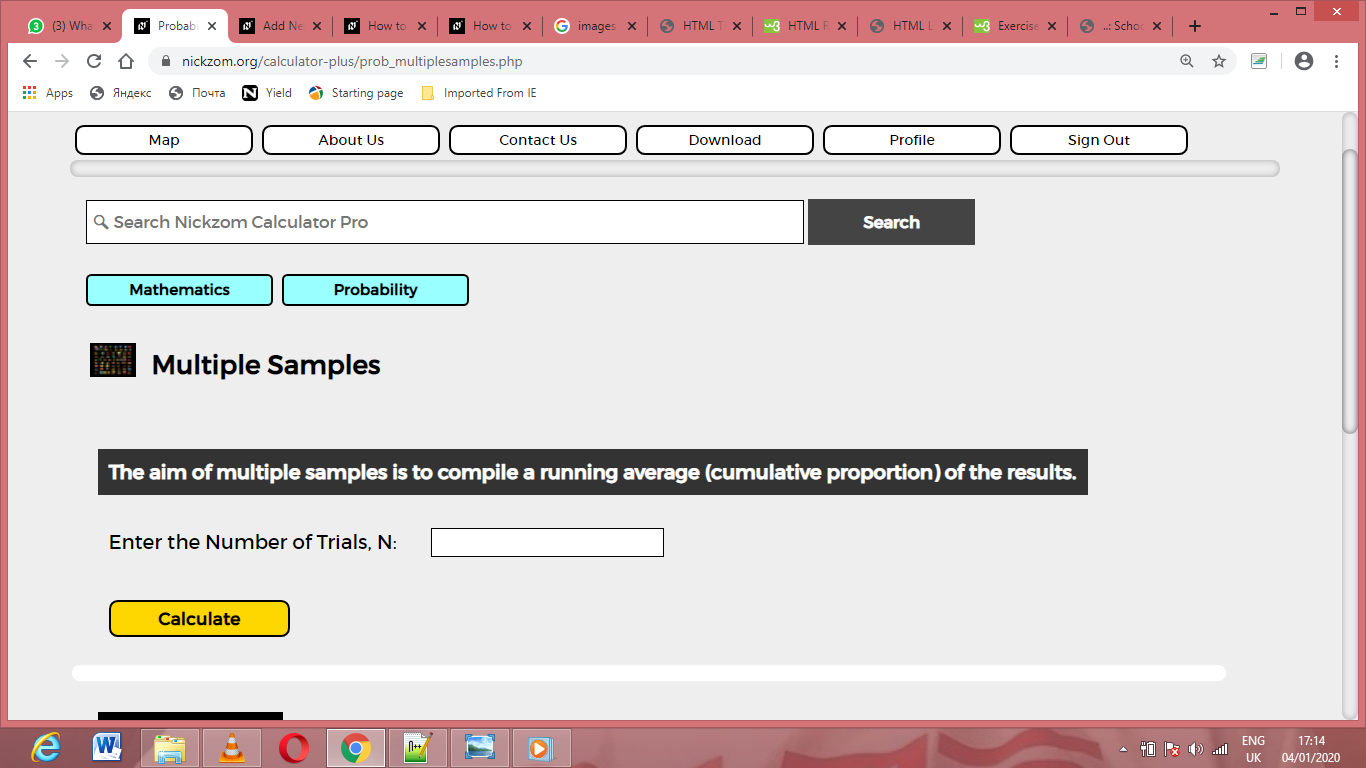Now, enter the value appropriately and accordingly for the parameter as required by the Number of Trial (N) is 4.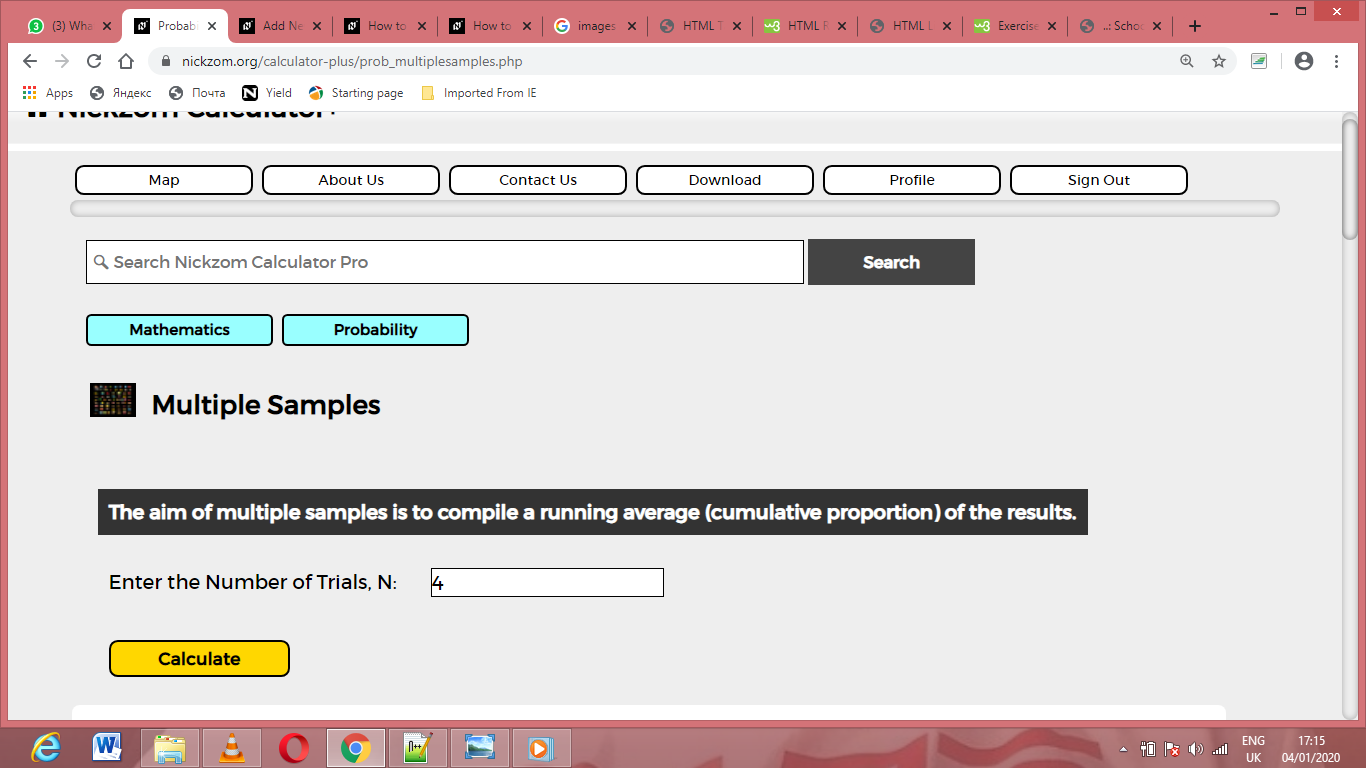Now, enter the number of successes x in each trialFinally, Click on Calculate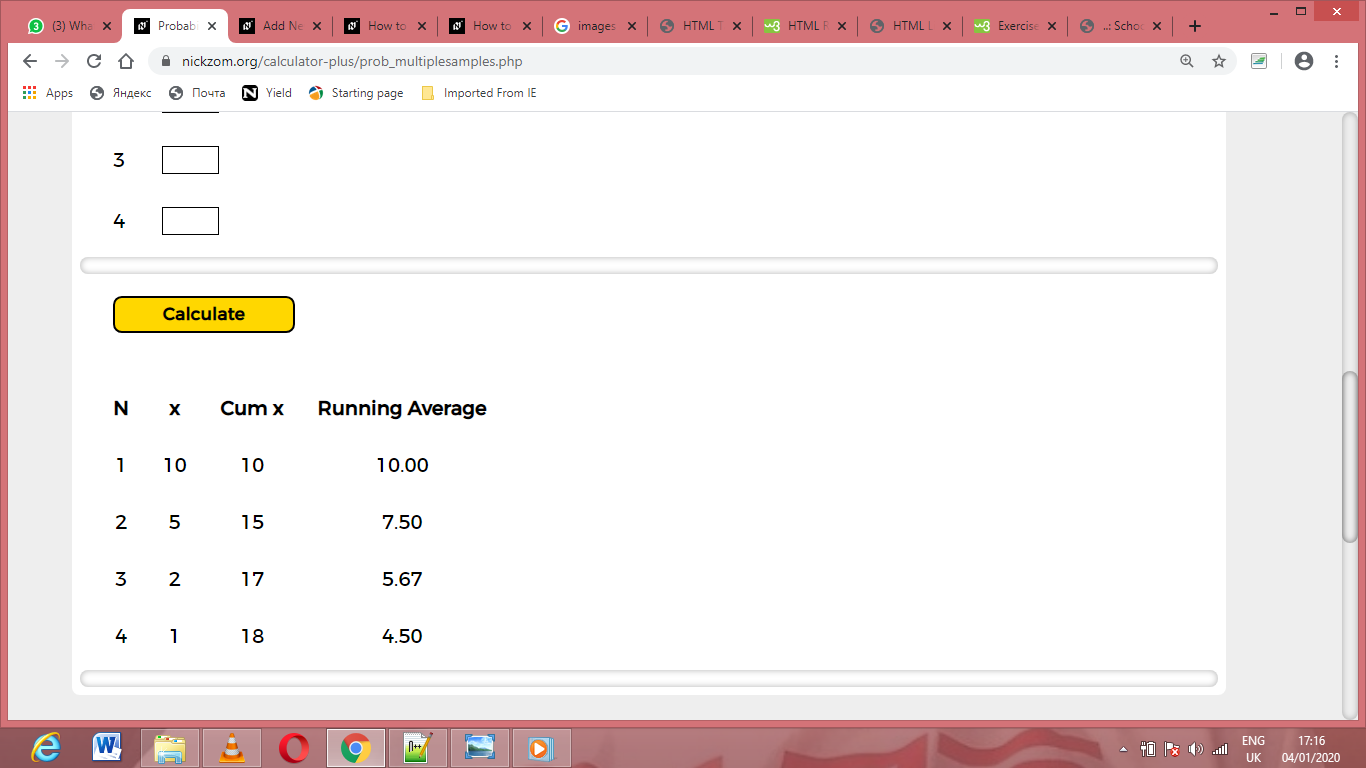As you can see from the screenshot above, Nickzom Calculator– The Calculator Encyclopedia solves for the multiple samples, presents the formula, workings and steps too.

## How to Calculate and Solve for Success and Failure | Probability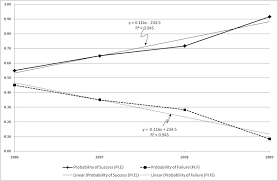The image above represents success and failure.

To compute for success and failure, two essential parameters are needed and these parameters are and N.

The formula for calculating success:

P(success) = x N

Where;

x = Number of successes
N = Total Number of Trials or Items

Let’s solve an example;
Find the success when the x is 12 and N is 14?

This implies that;

x = Number of successes = 12
N = Total Number of Trials or Items = 14

P(success) = x N
P(success) = 12 14
Dividing the numerator and denominator by 2
P(success) = 6 7
P(success) = 0.857

Therefore, the success is 0.857.

## How to Calculate and Solve for Expectation | Probability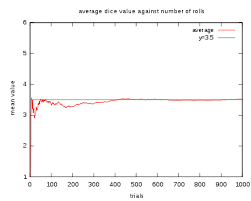The image above represents expectation.

To compute for expectation, two essential parameters are needed and these parameters are Number of Items or Trials (N) and Probability that the Event A will occur in any one Trial, P(A).

The formula for calculating expectation:

Expectation = N x P(A)

Where;

N = Number of Item or Trial
P(A) = Probability that the Event A will occur in any one Trial

Let’s solve an example;
Find the expectation when the number of items is 20 and the probability that the event A will occur in any one trial is 1.

This implies that;

N = Number of Item or Trial = 20
P(A) = Probability that the Event A will occur in any one Trial = 1

Expectation = N x P(A)
Expectation = 20 x 1
Expectation = 20

Therefore, the expectation is 20.

Calculating for Number of Items or Trial when the Expectation and the Probability that the Event A will occur in any one Trial is Given.

N = Expectation / P(A)

Where;

N = Number of Items or Trial
P(A) = Probability that the Event A will occur in any one Trial

Let’s solve an example;
Find the number of items or trial when the expectation is 25 and the probability that the Event A will occur in any one Trial is 1.

This implies that;

Expectation = 25
P(A) = Probability that the Event A will occur in any one Trial = 1

N = Expectation / P(A)
N = 25 / 1
N = 25

Therefore, the number of items or trial is 25.